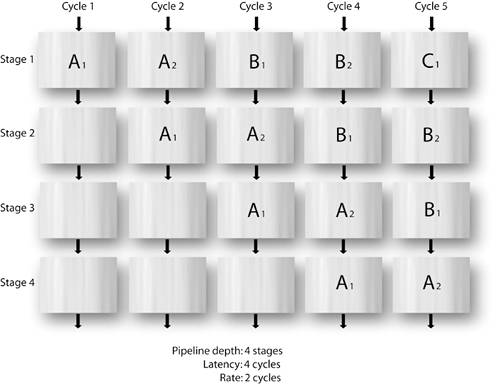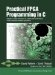# Section 7.6. Pipelining Explained

### 7.6. Pipelining Explained

When pipelining is enabled for inner code loops of your application (through the use of the PIPELINE pragma), the Impulse optimizer attempts to parallelize statements appearing within that loop with the goal of reducing the number of instruction cycles required to process the entire pipeline.

Pipelining is conceptually similar to a manufacturing assembly line, where the person at the first station of the assembly line can send a product to the next station for more assembly while he or she starts working on a second product. In this example, each station performs a portion of the overall assembly work. In a hardware pipeline, the body of a loop containing a sequence of operations is divided into stages that are analogous to the stations of the assembly line. Each stage performs a portion of the loop body. After the first stage has completed its portion of loop iteration i, the second stage begins its portion of iteration i, while the first stage starts processing iteration i+1 in parallel.

Pipelining reduces the number of cycles required to execute a loop by allowing the operations of one iteration to execute in parallel with operations of one or more subsequent iterations. Figure 7-2 illustrates a four-stage pipeline that is operating on three data packets (A, B, and C), each of which requires four clock cycles for the complete computation. By introducing a pipeline, the effective throughput rate of the process can be increased as shown. In this example it is assumed that each data packet requires two sequential operations (two pipeline stages) before proceeding to the next computation, resulting in an effective throughput rate of two cycles per data packet, which is an effective doubling of performance. Other pipelines may have greater or lesser performance gains, depending on the number of dependencies between different pipeline stages and other factors.

##### Figure 7-2. A four-stage pipeline with a throughput rate of two cycles.For a more concrete example, consider the following loop:

` while (1) {   if (co_stream_read(istream,&data,sizeof(data)) != co_err_none)       break;   sum += data;   co_stream_write(ostream,&sum,sizeof(sum)); } `

Without pipelining, the body of this loop requires two cycles: the first cycle reads data from the input stream, and the second performs the addition and writes the result to the output stream. This example requires 200 cycles to process 100 inputs. Now, suppose that pipelining were used:

` while (1) { #pragma CO PIPELINE   if (co_stream_read(istream,&data,sizeof(data)) != co_err_none)       break;   sum += data;   co_stream_write(ostream,&sum,sizeof(sum)); } `

This example results in a pipeline with two stages. The first stage reads data from the input stream, and the second stage performs the addition and writes the result to the output stream, similar to before. In the pipelined version, however, after the first data value is read, stage one immediately starts reading the next input in parallel with the computation and output of the sum using the first input. This example now requires only 101 cycles to process 100 inputs, or about one cycle per iteration.

The number of cycles required to complete one iteration of the loop is equal to the number of stages in the pipeline and is usually called the latency of the pipeline. In this example the latency is two.

#### Pipeline Rate

In most cases it is not possible to perform all stages of a pipeline in parallel. This can occur, for example, if two stages read from the same memory, such as from a local array. As a result, the pipeline will not be able to complete an iteration of the loop every cycle. The pipeline rate is the number of cycles between the time an iteration begins execution and the time the next iteration is allowed to begin. (This is sometimes called the input rate or introduction rate.) For example, if the rate were two, the pipeline would require about two times the number of iterations to complete the loop.

The fastest rate at which a loop can execute depends on inter-iteration dependencies and the use of sequential resources such as memories and streams. A common cause of reduced pipeline rates is multiple loads/stores of the same array variable.

The rate of a pipeline that contains multiple loads/stores of a single multidimensional array can sometimes be improved by using multiple arrays. For example, an image stored as RGB values might be implemented in C as

` int8 img; `

This array has three columns for the red, blue, and green components of each pixel. If img[i], img[i], and img[i] all appeared in the loop, the pipeline rate would be increased to permit three reads from the img array.

To improve performance, the image array might be repartitioned as follows:

` int8 red,green,blue; `

The loop body now references red[i], green[i], and blue[i]. Because these are separate arrays, they can be read simultaneously, and the pipeline rate will be improved.Practical FPGA Programming in C
ISBN: 0131543180
EAN: 2147483647
Year: 2005
Pages: 208

Similar book on Amazon